# 6.4 Graphs of logarithmic functions  (Page 8/8)

 Page 8 / 8

## Verbal

The inverse of every logarithmic function is an exponential function and vice-versa. What does this tell us about the relationship between the coordinates of the points on the graphs of each?

Since the functions are inverses, their graphs are mirror images about the line $\text{\hspace{0.17em}}y=x.\text{\hspace{0.17em}}$ So for every point $\text{\hspace{0.17em}}\left(a,b\right)\text{\hspace{0.17em}}$ on the graph of a logarithmic function, there is a corresponding point $\text{\hspace{0.17em}}\left(b,a\right)\text{\hspace{0.17em}}$ on the graph of its inverse exponential function.

What type(s) of translation(s), if any, affect the range of a logarithmic function?

What type(s) of translation(s), if any, affect the domain of a logarithmic function?

Shifting the function right or left and reflecting the function about the y-axis will affect its domain.

Consider the general logarithmic function $\text{\hspace{0.17em}}f\left(x\right)={\mathrm{log}}_{b}\left(x\right).\text{\hspace{0.17em}}$ Why can’t $\text{\hspace{0.17em}}x\text{\hspace{0.17em}}$ be zero?

Does the graph of a general logarithmic function have a horizontal asymptote? Explain.

No. A horizontal asymptote would suggest a limit on the range, and the range of any logarithmic function in general form is all real numbers.

## Algebraic

For the following exercises, state the domain and range of the function.

$f\left(x\right)={\mathrm{log}}_{3}\left(x+4\right)$

$h\left(x\right)=\mathrm{ln}\left(\frac{1}{2}-x\right)$

Domain: $\text{\hspace{0.17em}}\left(-\infty ,\frac{1}{2}\right);\text{\hspace{0.17em}}$ Range: $\text{\hspace{0.17em}}\left(-\infty ,\infty \right)$

$g\left(x\right)={\mathrm{log}}_{5}\left(2x+9\right)-2$

$h\left(x\right)=\mathrm{ln}\left(4x+17\right)-5$

Domain: $\text{\hspace{0.17em}}\left(-\frac{17}{4},\infty \right);\text{\hspace{0.17em}}$ Range: $\text{\hspace{0.17em}}\left(-\infty ,\infty \right)$

$f\left(x\right)={\mathrm{log}}_{2}\left(12-3x\right)-3$

For the following exercises, state the domain and the vertical asymptote of the function.

$\text{\hspace{0.17em}}f\left(x\right)={\mathrm{log}}_{b}\left(x-5\right)$

Domain: $\text{\hspace{0.17em}}\left(5,\infty \right);\text{\hspace{0.17em}}$ Vertical asymptote: $\text{\hspace{0.17em}}x=5$

$\text{\hspace{0.17em}}g\left(x\right)=\mathrm{ln}\left(3-x\right)$

$\text{\hspace{0.17em}}f\left(x\right)=\mathrm{log}\left(3x+1\right)$

Domain: $\text{\hspace{0.17em}}\left(-\frac{1}{3},\infty \right);\text{\hspace{0.17em}}$ Vertical asymptote: $\text{\hspace{0.17em}}x=-\frac{1}{3}$

$\text{\hspace{0.17em}}f\left(x\right)=3\mathrm{log}\left(-x\right)+2$

$\text{\hspace{0.17em}}g\left(x\right)=-\mathrm{ln}\left(3x+9\right)-7$

Domain: $\text{\hspace{0.17em}}\left(-3,\infty \right);\text{\hspace{0.17em}}$ Vertical asymptote: $\text{\hspace{0.17em}}x=-3$

For the following exercises, state the domain, vertical asymptote, and end behavior of the function.

$f\left(x\right)=\mathrm{ln}\left(2-x\right)$

$f\left(x\right)=\mathrm{log}\left(x-\frac{3}{7}\right)$

Domain: $\left(\frac{3}{7},\infty \right)$ ;
Vertical asymptote: $x=\frac{3}{7}$ ; End behavior: as $x\to {\left(\frac{3}{7}\right)}^{+},f\left(x\right)\to -\infty$ and as $x\to \infty ,f\left(x\right)\to \infty$

$h\left(x\right)=-\mathrm{log}\left(3x-4\right)+3$

$g\left(x\right)=\mathrm{ln}\left(2x+6\right)-5$

Domain: $\left(-3,\infty \right)$ ; Vertical asymptote: $x=-3$ ;
End behavior: as $x\to -{3}^{+}$ , $f\left(x\right)\to -\infty$ and as $x\to \infty$ , $f\left(x\right)\to \infty$

$f\left(x\right)={\mathrm{log}}_{3}\left(15-5x\right)+6$

For the following exercises, state the domain, range, and x - and y -intercepts, if they exist. If they do not exist, write DNE.

$h\left(x\right)={\mathrm{log}}_{4}\left(x-1\right)+1$

Domain: $\text{\hspace{0.17em}}\left(1,\infty \right);\text{\hspace{0.17em}}$ Range: $\text{\hspace{0.17em}}\left(-\infty ,\infty \right);\text{\hspace{0.17em}}$ Vertical asymptote: $\text{\hspace{0.17em}}x=1;\text{\hspace{0.17em}}$ x -intercept: $\text{\hspace{0.17em}}\left(\frac{5}{4},0\right);\text{\hspace{0.17em}}$ y -intercept: DNE

$f\left(x\right)=\mathrm{log}\left(5x+10\right)+3$

$g\left(x\right)=\mathrm{ln}\left(-x\right)-2$

Domain: $\text{\hspace{0.17em}}\left(-\infty ,0\right);\text{\hspace{0.17em}}$ Range: $\text{\hspace{0.17em}}\left(-\infty ,\infty \right);\text{\hspace{0.17em}}$ Vertical asymptote: $\text{\hspace{0.17em}}x=0;\text{\hspace{0.17em}}$ x -intercept: $\text{\hspace{0.17em}}\left(-{e}^{2},0\right);\text{\hspace{0.17em}}$ y -intercept: DNE

$f\left(x\right)={\mathrm{log}}_{2}\left(x+2\right)-5$

$h\left(x\right)=3\mathrm{ln}\left(x\right)-9$

Domain: $\text{\hspace{0.17em}}\left(0,\infty \right);\text{\hspace{0.17em}}$ Range: $\text{\hspace{0.17em}}\left(-\infty ,\infty \right);\text{\hspace{0.17em}}$ Vertical asymptote: $\text{\hspace{0.17em}}x=0;\text{\hspace{0.17em}}$ x -intercept: $\text{\hspace{0.17em}}\left({e}^{3},0\right);\text{\hspace{0.17em}}$ y -intercept: DNE

## Graphical

For the following exercises, match each function in [link] with the letter corresponding to its graph.

$d\left(x\right)=\mathrm{log}\left(x\right)$

$f\left(x\right)=\mathrm{ln}\left(x\right)$

B

$g\left(x\right)={\mathrm{log}}_{2}\left(x\right)$

$h\left(x\right)={\mathrm{log}}_{5}\left(x\right)$

C

$j\left(x\right)={\mathrm{log}}_{25}\left(x\right)$

For the following exercises, match each function in [link] with the letter corresponding to its graph.

$f\left(x\right)={\mathrm{log}}_{\frac{1}{3}}\left(x\right)$

B

$g\left(x\right)={\mathrm{log}}_{2}\left(x\right)$

$h\left(x\right)={\mathrm{log}}_{\frac{3}{4}}\left(x\right)$

C

For the following exercises, sketch the graphs of each pair of functions on the same axis.

$f\left(x\right)=\mathrm{log}\left(x\right)\text{\hspace{0.17em}}$ and $\text{\hspace{0.17em}}g\left(x\right)={10}^{x}$

$f\left(x\right)=\mathrm{log}\left(x\right)\text{\hspace{0.17em}}$ and $\text{\hspace{0.17em}}g\left(x\right)={\mathrm{log}}_{\frac{1}{2}}\left(x\right)$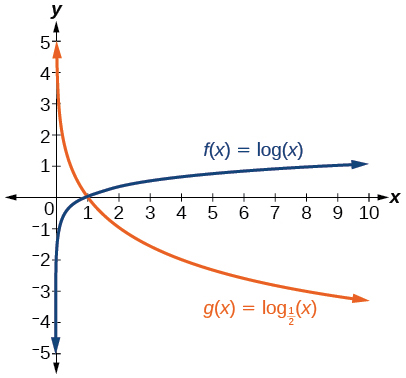$f\left(x\right)={\mathrm{log}}_{4}\left(x\right)\text{\hspace{0.17em}}$ and $\text{\hspace{0.17em}}g\left(x\right)=\mathrm{ln}\left(x\right)$

$f\left(x\right)={e}^{x}\text{\hspace{0.17em}}$ and $\text{\hspace{0.17em}}g\left(x\right)=\mathrm{ln}\left(x\right)$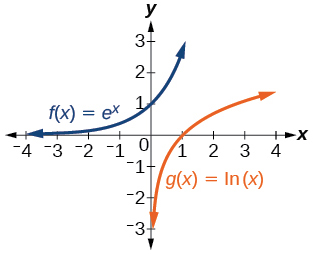For the following exercises, match each function in [link] with the letter corresponding to its graph.

$f\left(x\right)={\mathrm{log}}_{4}\left(-x+2\right)$

$g\left(x\right)=-{\mathrm{log}}_{4}\left(x+2\right)$

C

$h\left(x\right)={\mathrm{log}}_{4}\left(x+2\right)$

For the following exercises, sketch the graph of the indicated function.

$\text{\hspace{0.17em}}f\left(x\right)={\mathrm{log}}_{2}\left(x+2\right)$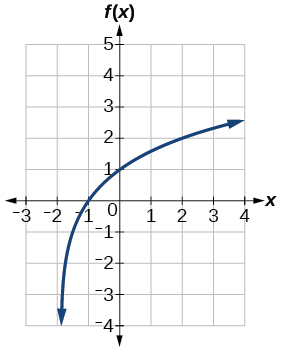$\text{\hspace{0.17em}}f\left(x\right)=2\mathrm{log}\left(x\right)$

$\text{\hspace{0.17em}}f\left(x\right)=\mathrm{ln}\left(-x\right)$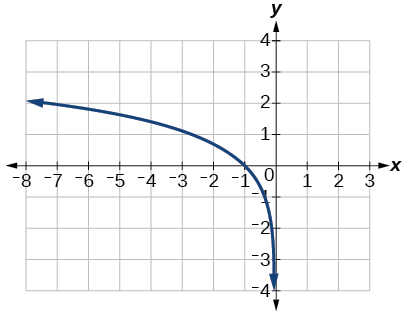$g\left(x\right)=\mathrm{log}\left(4x+16\right)+4$

$g\left(x\right)=\mathrm{log}\left(6-3x\right)+1$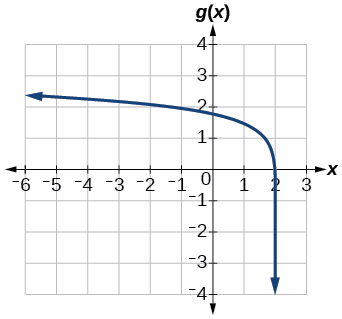$h\left(x\right)=-\frac{1}{2}\mathrm{ln}\left(x+1\right)-3$

For the following exercises, write a logarithmic equation corresponding to the graph shown.

Use $\text{\hspace{0.17em}}y={\mathrm{log}}_{2}\left(x\right)\text{\hspace{0.17em}}$ as the parent function.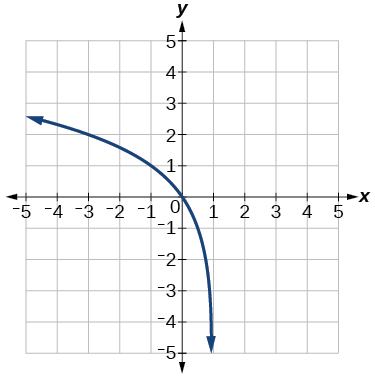$\text{\hspace{0.17em}}f\left(x\right)={\mathrm{log}}_{2}\left(-\left(x-1\right)\right)$

Use $\text{\hspace{0.17em}}f\left(x\right)={\mathrm{log}}_{3}\left(x\right)\text{\hspace{0.17em}}$ as the parent function.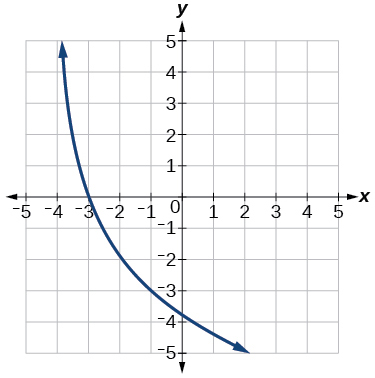Use $\text{\hspace{0.17em}}f\left(x\right)={\mathrm{log}}_{4}\left(x\right)\text{\hspace{0.17em}}$ as the parent function.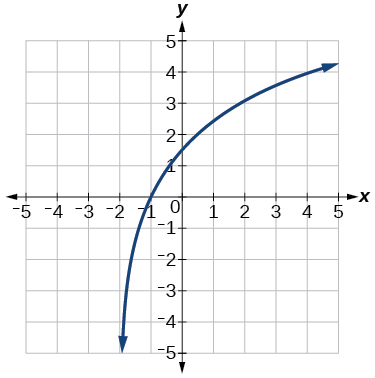$f\left(x\right)=3{\mathrm{log}}_{4}\left(x+2\right)$

Use $\text{\hspace{0.17em}}f\left(x\right)={\mathrm{log}}_{5}\left(x\right)\text{\hspace{0.17em}}$ as the parent function.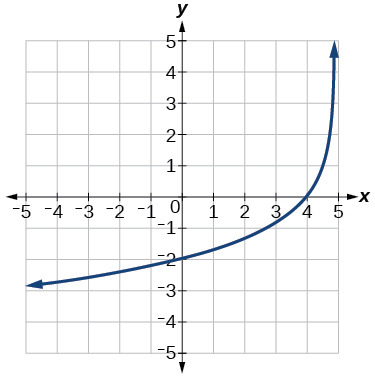## Technology

For the following exercises, use a graphing calculator to find approximate solutions to each equation.

$\mathrm{log}\left(x-1\right)+2=\mathrm{ln}\left(x-1\right)+2$

$x=2$

$\mathrm{log}\left(2x-3\right)+2=-\mathrm{log}\left(2x-3\right)+5$

$\mathrm{ln}\left(x-2\right)=-\mathrm{ln}\left(x+1\right)$

$x\approx \text{2}\text{.303}$

$2\mathrm{ln}\left(5x+1\right)=\frac{1}{2}\mathrm{ln}\left(-5x\right)+1$

$\frac{1}{3}\mathrm{log}\left(1-x\right)=\mathrm{log}\left(x+1\right)+\frac{1}{3}$

$x\approx -0.472$

## Extensions

Let $\text{\hspace{0.17em}}b\text{\hspace{0.17em}}$ be any positive real number such that $\text{\hspace{0.17em}}b\ne 1.\text{\hspace{0.17em}}$ What must $\text{\hspace{0.17em}}{\mathrm{log}}_{b}1\text{\hspace{0.17em}}$ be equal to? Verify the result.

Explore and discuss the graphs of $\text{\hspace{0.17em}}f\left(x\right)={\mathrm{log}}_{\frac{1}{2}}\left(x\right)\text{\hspace{0.17em}}$ and $\text{\hspace{0.17em}}g\left(x\right)=-{\mathrm{log}}_{2}\left(x\right).\text{\hspace{0.17em}}$ Make a conjecture based on the result.

The graphs of $\text{\hspace{0.17em}}f\left(x\right)={\mathrm{log}}_{\frac{1}{2}}\left(x\right)\text{\hspace{0.17em}}$ and $\text{\hspace{0.17em}}g\left(x\right)=-{\mathrm{log}}_{2}\left(x\right)\text{\hspace{0.17em}}$ appear to be the same; Conjecture: for any positive base $\text{\hspace{0.17em}}b\ne 1,$ $\text{\hspace{0.17em}}{\mathrm{log}}_{b}\left(x\right)=-{\mathrm{log}}_{\frac{1}{b}}\left(x\right).$

Prove the conjecture made in the previous exercise.

What is the domain of the function $\text{\hspace{0.17em}}f\left(x\right)=\mathrm{ln}\left(\frac{x+2}{x-4}\right)?\text{\hspace{0.17em}}$ Discuss the result.

Recall that the argument of a logarithmic function must be positive, so we determine where $\text{\hspace{0.17em}}\frac{x+2}{x-4}>0\text{\hspace{0.17em}}$ . From the graph of the function $\text{\hspace{0.17em}}f\left(x\right)=\frac{x+2}{x-4},$ note that the graph lies above the x -axis on the interval $\text{\hspace{0.17em}}\left(-\infty ,-2\right)\text{\hspace{0.17em}}$ and again to the right of the vertical asymptote, that is $\text{\hspace{0.17em}}\left(4,\infty \right).\text{\hspace{0.17em}}$ Therefore, the domain is $\text{\hspace{0.17em}}\left(-\infty ,-2\right)\cup \left(4,\infty \right).$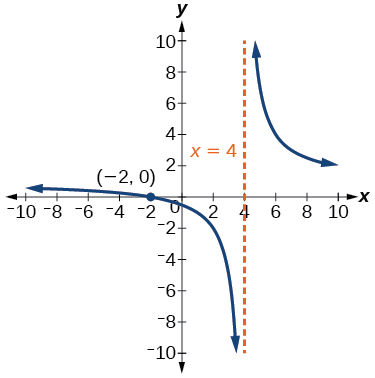Use properties of exponents to find the x -intercepts of the function $\text{\hspace{0.17em}}f\left(x\right)=\mathrm{log}\left({x}^{2}+4x+4\right)\text{\hspace{0.17em}}$ algebraically. Show the steps for solving, and then verify the result by graphing the function.

A laser rangefinder is locked on a comet approaching Earth. The distance g(x), in kilometers, of the comet after x days, for x in the interval 0 to 30 days, is given by g(x)=250,000csc(π30x). Graph g(x) on the interval [0, 35]. Evaluate g(5)  and interpret the information. What is the minimum distance between the comet and Earth? When does this occur? To which constant in the equation does this correspond? Find and discuss the meaning of any vertical asymptotes.
The sequence is {1,-1,1-1.....} has
how can we solve this problem
Sin(A+B) = sinBcosA+cosBsinA
Prove it
Eseka
Eseka
hi
Joel
June needs 45 gallons of punch. 2 different coolers. Bigger cooler is 5 times as large as smaller cooler. How many gallons in each cooler?
7.5 and 37.5
Nando
find the sum of 28th term of the AP 3+10+17+---------
I think you should say "28 terms" instead of "28th term"
Vedant
the 28th term is 175
Nando
192
Kenneth
if sequence sn is a such that sn>0 for all n and lim sn=0than prove that lim (s1 s2............ sn) ke hole power n =n
write down the polynomial function with root 1/3,2,-3 with solution
if A and B are subspaces of V prove that (A+B)/B=A/(A-B)
write down the value of each of the following in surd form a)cos(-65°) b)sin(-180°)c)tan(225°)d)tan(135°)
Prove that (sinA/1-cosA - 1-cosA/sinA) (cosA/1-sinA - 1-sinA/cosA) = 4
what is the answer to dividing negative index
In a triangle ABC prove that. (b+c)cosA+(c+a)cosB+(a+b)cisC=a+b+c.
give me the waec 2019 questionsByByByByByByBy